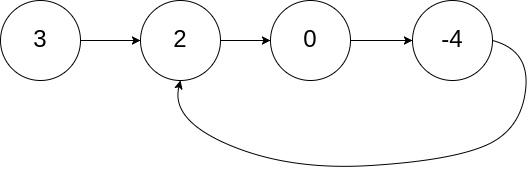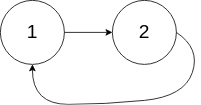# 142. 环形链表 II

## 问题## 解法

• 慢指针再走$$a+b$$得距离就回到$$B$$点
• 慢指针再走$$a$$的距离就到了$$A$$点
第一点对我们解本题没有什么用，看第二点，我们在一开始定义的$$a$$就是从$$head$$到入环点$$A$$的距离，那么我们可以将快指针重置到$$head$$处，然后快慢指针同时前进，当快慢指针相等时，就是入环点，返回即可。

## 代码

### java实现

/**
* class ListNode {
*     int val;
*     ListNode next;
*     ListNode(int x) {
*         val = x;
*         next = null;
*     }
* }
*/
public class Solution {
return null;
}
//使用快慢指针的方式进行
while (fast != null && fast.next != null && fast.next.next != null) {
fast = fast.next.next;
slow = slow.next;
if (fast == slow) {
//此时说明有环，需要处理
while(true) {
if (slow == fast) {
return slow;
}
slow = slow.next;
fast = fast.next;
}
}
}
//无环状态
return null;
}
}